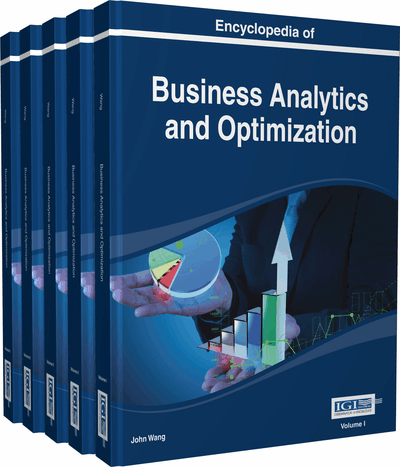# Randomizing DEA Efficiency Scores with Beta Distribution

P. Sunil Dharmapala (College of Economics & Political Science, Sultan Qaboos University, Sultanate of Oman)
DOI: 10.4018/978-1-4666-5202-6.ch178

## Abstract

A criticism leveled against Data Envelopment Analysis (DEA) is that it is incapable of handling input/output data contaminated with random errors, and therefore, efficiency scores reported by DEA do not reflect reality. Several researchers have addressed this issue by incorporating statistical noise into DEA modeling, thus giving birth to Stochastic DEA. In this chapter, utilizing well known DEA models, we propose a method to randomize efficiency scores by treating each score as an order statistic of an underlying Beta distribution. In an application to a set of banks, we demonstrate how to do this randomization and derive some statistical results.
Chapter Preview
Top

## Literature Review

A comprehensive listing and analysis of DEA research that covers the first 30 years of its history could be found in Emrouznejad et al. (2008). In this chapter, we narrow down our literature survey to stochastic DEA modeling, as it suits the theme of discussion of the chapter.

DEA measures relative efficiency of decision making units (DMUs), assuming that input/output data are deterministic. While highlighting this as a drawback in DEA methodology, some authors in the past incorporated statistical noise into DEA modelling and berthed Stochastic DEA (SDEA). Some of them treated the input/output vectors as independent and jointly “multivariate normal” random vectors, whose components are expected values of input/outputs. Thus, each input/output of each DMU was treated as a “normally distributed” random variable. Then, the constraints on inputs and outputs (in the traditional DEA model) were expressed in probabilistic terms with the noise parameter (degree of uncertainty) attached to them. Some others treated inputs/outputs as “means” of series of observations and constructed “confidence intervals” for the means.

Cooper et al. (1998, 2002a, 2004), Despotis and Smirlis (2002), Gstach (1998), Huang and Li (1996), Kao (2006), Olesen and Petersen (1995), and Sengupta (1987, 1998), are among them. Simar and Wilson (1998) used the “bootstrap method” in Statistics in the sensitivity analysis of efficiency scores. This method goes by creating many samples (bootstrap samples) from an original sample of efficiency scores and constructing interval estimates for the efficiency scores from those samples, with “bootstrap percentiles” reflecting the degree of confidence through high probability.

Top

## Methodology

Thompson et al. (1993) presented a new DEA theory, which did not require the use of non-Archimedean principle used in the original DEA theory developed by Charnes et al. (1978), and it allowed zero data entries. In this paper, we use Thompson et al.’s methodology as described below.

A DEA data domain consists of n decision-making units (DMUs), n input vectors (each with m inputs), and n output vectors (each with r outputs). The selected DMUc (c = 1, 2,…, n) is characterized by an input vector Xc (x1c, x2c, …, xmc) and an output vector Yc (y1c, y2c, …, yrc). U-output multiplier of r unknowns (uk ; k = 1, 2,…,r ) and V-input multiplier of m unknowns (vi ; i = 1, 2,…m) have to be determined by solving the respective linear programming (LP) models stated below.

Here we consider the following four inputs (m = 4) and two outputs (r = 2) for the banks:

## Key Terms in this Chapter

Multipliers: Weights v i on inputs and u k on outputs that need to be determined by solving the linear programming model CCR/AR or BCC/AR.

Price/Cost Data: The range of values of multipliers defined by the ratio of Revenue-to-Cost using the market prices.

Input-Oriented Model: Find the best set of weights to maximize the weighted ratio of outputs-to-inputs at fixed levels of inputs, subject to the constraint that no ratio is more than unity.

Efficient DMU: A DMU with maximum Z-score 1, provided that all input and output slacks in the optimal solution (in CCR/AR or BCC/AR model) are zero. (If max Z < 1, the DMU is inefficient).

Order Statistics: A sample of random variables that are arranged in increasing order, from the smallest to the largest.

Assurance Regions: Restrictions on input-multipliers and output-multipliers in the form of constraints (3) and (4) that lead to the construction of input-cone and output-cone respectively, in CCR/AR and BCC/AR models.

Constant Returns: Neither (i) nor (ii) in variable returns.

Variable Returns: For a fixed DMU c with input-output vector (X c , Y c ), (i) it is possible to achieve a more than proportionate increment in Y c when X c is augmented (Increasing returns), or (ii) it is possible to achieve a less than proportionate decrement in Y c when X c is decremented (Decreasing returns).

## Complete Chapter List

Search this Book:
Reset